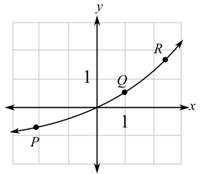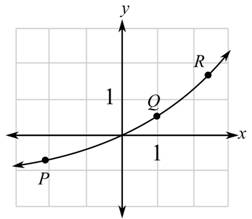Chapter 10.5, Problem 17EFinite Mathematics and Applied Cal...

7th Edition
Stefan Waner + 1 other
ISBN: 9781337274203

Solutions

Chapter
SectionFinite Mathematics and Applied Cal...

7th Edition
Stefan Waner + 1 other
ISBN: 9781337274203
Textbook Problem

In Exercises 17–22, say at which labeled point the slope of the tangent is (a) greatest and (b) least (in the sense that − 7 is less than 1). [HINT: See Quick Example 3.](a)

To determine

The greatest slope of the tangent among the labeled points in the graph which is as follows:Explanation

Given information:

The provided graph is:

When a function is increasing or rising the slope of the function is greatest where the tangent is more slanted whereas when the function is decreasing or falling it has the least value where the tangent is the most slanted.

From the graph, one can observe that the function is rising...

(b)

To determine

The least slope of the tangent among the labeled points in the graph which is as follows:Still sussing out bartleby?

Check out a sample textbook solution.

See a sample solution

The Solution to Your Study Problems

Bartleby provides explanations to thousands of textbook problems written by our experts, many with advanced degrees!

Get Started

Change 0.35 A to mA.

Elementary Technical Mathematics

In problems 15-22, simplify by combining like terms. 16.

Mathematical Applications for the Management, Life, and Social Sciences

Evaluate the limit. limx0tan4xx+sin2x

Single Variable Calculus: Early Transcendentals

For 0

Study Guide for Stewart's Multivariable Calculus, 8th

n=12(14)n converges to: a) 94 b) 2 c) 23 d) the series diverges

Study Guide for Stewart's Single Variable Calculus: Early Transcendentals, 8th